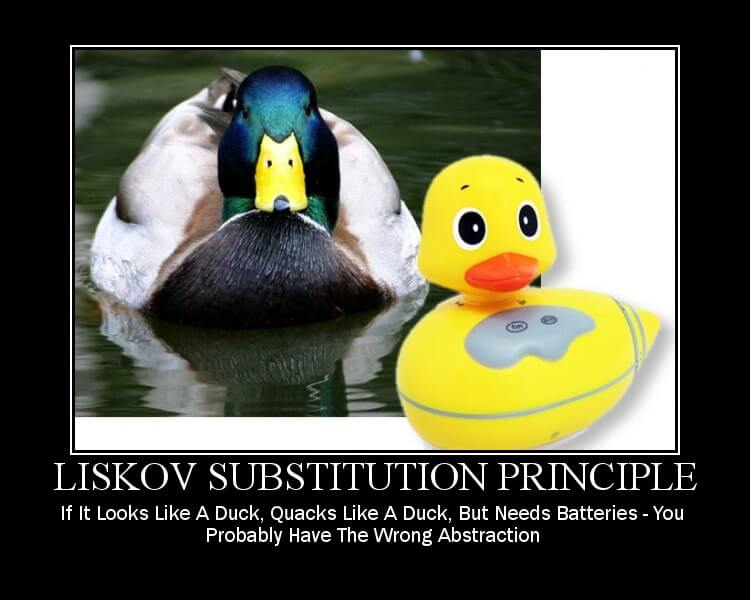## What Is This Principle?

The Liskov Substitution Principle(LSP), named for and originally defined by Barbara Liskov, states that we should be able to treat a child class as though it were the parent class. Essentially this means that all derived classes should retain the functionality of their parent class and cannot replace any functionality the parent provides. The LSP is very similar in principle to the Open/Closed Principle.## Benefits

This principle aims to keep functionality intact. It's main purpose is to guarantee that objects lower in a relational hierarchy can be treated as though they are objects higher in the hierarchy. Basically, any child class should be able to do anything the parent can do.

## A Simple Example

We'll use the classic Circle-Ellipse problem to demonstrate this principle. Let's imagine that we need to find the area of any ellipse. So, we create a class that represents an ellipse:

``````public class Ellipse
{
public double MajorAxis { get; set; }
public double MinorAxis { get; set; }

public virtual void SetMajorAxis(double majorAxis)
{
MajorAxis = majorAxis;
}

public virtual void SetMinorAxis(double minorAxis)
{
MinorAxis = minorAxis;
}

public virtual double Area()
{
return MajorAxis * MinorAxis * Math.PI;
}
}
``````

We know from high school geometry that a circle is just a special case for an ellipse, so we create a Circle class that inherits from Ellipse, but SetMajorAxis sets both axes (because in a circle, the major and minor axes must always be the same, which is just the radius):

``````public class Circle : Ellipse
{
public override void SetMajorAxis(double majorAxis)
{
base.SetMajorAxis(majorAxis);
this.MinorAxis = majorAxis; //In a cirle, each axis is identical
}
}
``````

See the problem now? If we set both axes, attempting to calculate the area gives the wrong result.

``````Circle circle = new Circle();
circle.SetMajorAxis(5);
circle.SetMinorAxis(4);
var area = circle.Area(); //5*4 = 20, but we expected 5*5 = 25
``````

This is a violation of the Liskov Substitution Principle. However, the best way to refactor this code is not obvious, as there are quite a few possibilities. One solution might be to have Circle implement SetMinorAxis as well:

``````public class Circle : Ellipse
{
public override void SetMajorAxis(double majorAxis)
{
base.SetMajorAxis(majorAxis);
this.MinorAxis = majorAxis; //In a cirle, each axis is identical
}

public override void SetMinorAxis(double minorAxis)
{
base.SetMinorAxis(minorAxis);
this.MajorAxis = minorAxis;
}

public override double Area()
{
return base.Area();
}
}
``````

Another solution, one with less code overall, might be to treat Circle as an entirely separate class:

``````public class Circle
{
public double Radius { get; set; }
{
}

public double Area()
{